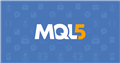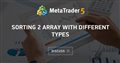# Sort array

I want to sort a dynamic array which has value in "double" in either ascending or descending order

When i use ArraySort function it returns invalid values so looking forward for any other method

Arpit T: I want to sort a dynamic array which has value in "double" in either ascending or descending order. When i use ArraySort function it returns invalid values so looking forward for any other method

You already know the drill. Show us your code so that so that we can help resolve your issue. Show also the output in the log.

Fernando Carreiro #:

You already know the drill. Show us your code so that so that we can help resolve your issue. Show also the output in the log.

```#define TOTAL 5

double vol[TOTAL]= {5,2,4,3,1};
string symbol[TOTAL]= {"A","B","C","D","E"};
//+------------------------------------------------------------------+
//| Script program start function                                    |
//+------------------------------------------------------------------+
void OnStart() {
//---
ArraySort(vol);

for(int i=0; i<TOTAL; i++) {

Print(symbol[i]+" "+vol[i]);
}
}```

i want to sort in descending order so 5,4,3,2,1 and also want to make sure symbol names are sorted with vol in output

Arpit T #:i want to sort in descending order so 5,4,3,2,1 and also want to make sure symbols are linked with vol in output

ArraySort only does ascending order and sorting the "vol" array does not affect the "symbol" array.

If you want them linked, then create a array of structure with both values and then create a sorting method or function for it.

You can also use the Data Collections from the Standard Library, for example.Documentation on MQL5: Standard Library / Data Collections
• www.mql5.com
Data Collections - Standard Library - MQL5 Reference - Reference on algorithmic/automated trading language for MetaTrader 5

Arpit T #:

i want to sort in descending order so 5,4,3,2,1 and also want to make sure symbol names are sorted with vol in output

You can use a 2d array and it will sort based on the first index of the 2nd dimension which allows you to keep the index of the symbol on the on the second index .

Then reverse the array and you get the descending order .

```#define TOTAL 5

double vol[];
double vol_values[]={5,2,4,3,1};
string symbol[]= {"A","B","C","D","E"};

void OnStart() {
//fill
ArrayResize(vol,TOTAL,0);
for(int i=0;i<TOTAL;i++){
vol[i]=vol_values[i];//the 0 index will be used in sorting which leaves the  index free
vol[i]=i;//which becomes the index reference
}

ArraySort(vol);
ArrayReverse(vol,0,TOTAL);//reverse for descending order

for(int i=0; i<TOTAL; i++) {
//get the reference ix to the symbol
int ix=(int)vol[i];
Print(symbol[ix]+" "+vol[i]);
}
}```

Here is a related topic with more information: Sorting 2 array with different types - How to sort multiple arrays?Sorting 2 array with different types - How to sort multiple arrays?
• 2019.09.05
• www.mql5.com
I wanna sort the array pipschange with the arraysort() function. Sorting one array has nothing to do with another. Then choose whether you want to use my way,           sort multiple arrays - mql4 programming forum

Lorentzos Roussos #:

You can use a 2d array and it will sort based on the first index of the 2nd dimension which allows you to keep the index of the symbol on the on the second index .

Then reverse the array and you get the descending order .

worked ! Thanks

Reason: